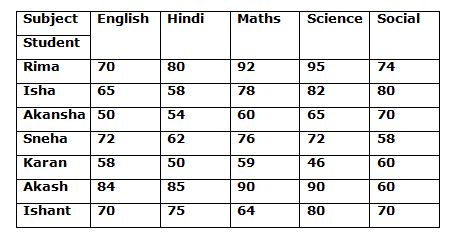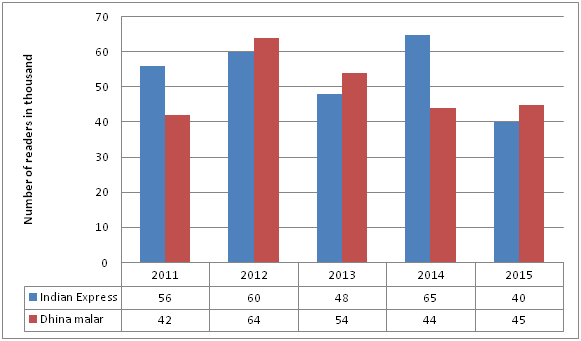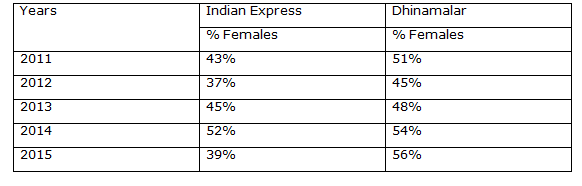# Quantitative Aptitude Questions (Data Interpretation) for IBPS RRB PO Prelims 2018 Day-165

Dear Readers, IBPS RRB is conducting Online Examination for the recruitment of probationary officer. To enrich your preparation here we have providing new series of Data Interpretation – Quantitative Aptitude Questions. Candidates those who are appearing in IBPS RRB PO Prelims Exams can practice these Quantitative Aptitude average questions daily and make your preparation effective.

Click “Start Quiz” to attend these Questions and view Solutions

Direction (1-5): Study the following information carefully and answer the following questions given below.

The table shows the percentage of marks obtained by different students in different subjects1) If maximum marks in Mathematics is 250, then what are the average marks scored by Rima, Isha, Akansha and Ishant together in mathematics?

a) 183.75

b) 120.75

c) 184.5

d) 165.75

e) 155.25

2) If the marks scored by Rima in Hindi and Social sciences are 160 and 222 respectively, then what is the ratio of marks scored by Isha in Hindi to the marks scored by Akansha in Social sciences?

a) 57: 105

b) 54: 103

c) 58: 105

d) 61: 103

e) 63: 105

3) If total marks in English are 200, then what is the average of marks scored by all 7 given persons in English?

a) 124

b) 154

c) 157

d) 134

e) 121

4) Total marks scored by Rima and Akansha in Mathematics are what percent of total marks scored by Karan and Akash in science?

a) 110%

b) 113.23%

c) 122.25%

d) 109.50%

e) Data insufficient.

5) If maximum marks in Science and Mathematics are equal, then what is the ratio of total marks scored by all 7 students in Science to the total marks scored by all 7 students in Mathematics?

a) 537:519

b) 530:523

c) 530:519

d) 523:511

e) 531:517

Directions (6-10): The following bar chart shows the total number of readers of newspaper Indian Express and Dhinamalar over the period of 2011 to 2015 and the table shows the percentage of female readers among them.6) What is the total number of female readers of newspaper Dhinamalar in the year 2014?

a) 24670

b) 33479

c) 23760

d) 43280

e) 25210

7) What is the difference between the total male readers of newspaper Indian Express and its total female readers in the year 2011?

a) 4570

b) 7840

c) 5670

d) 8280

e) 7560

8) What is the ratio of the total male readers of newspaper Indian Express in the year 2013 to the total female readers of newspaper Dhinamalar in the year 2015?

a) 22 : 21

b) 15: 22

c) 24: 13

d) 11 :43

e) 16: 15

9) What is the average number of male readers of newspaper Dhinamalar over the years?

a) 35470

b) 34650

c) 12460

d) 24780

e) 26760

10) The total number of female readers of newspaper Indian Express in the year 2012 is approximately what per cent of the total number of male readers of newspaper Dhinamalar in the year 2012?

a) 45%

b) 63%

c) 98%

d) 46%

e) 57%

Marks scored by Rima in Mathematics = (92/100) * 250 = 230

Marks scored by Isha in Mathematics = (78/100) * 250 = 195

Marks scored by Akansha in Mathematics = (60/100) * 250 = 150

Marks scored by Ishant in Mathematics = (64/100) * 250 = 160

Required average = (230 + 195 + 150 + 160)/4 = (735/4) =183.75

Let total marks in Hindi and Social sciences be H and S respectively.

Marks scored by Rima in Hindi = (80/100) * H

160 = (80/100) * H

H = 200.

Marks scored by Rima in Social sciences = (74/100) * S

222 = (74/100) * S

S = 300.

Marks scored by Isha in Hindi = (58/100) * 200 = 116.

Marks scored by Akansha in Social sciences = (70/100) * 300 = 210.

Required ratio = (116/210) = 58/105

Marks scored by Rima in English = (70/100) * 200 = 140

Marks scored by Isha in English = (65/100) * 200 = 130

Marks scored by Akansha in English = (50/100) * 200 = 100

Marks scored by Sneha in English = (72/100) * 200 = 144

Marks scored by Karan in English = (58/100) * 200 = 116

Marks scored by Akash in English = (84/100) * 200 = 168

Marks scored by Ishant in English = (70/100) * 200 = 140

Required average= (140 + 130 + 100 + 144 + 116 + 168 + 140)17

= (938/7) = 134.

As maximum marks of Science and Mathematics are not given so answer cannot be determined.

Let maximum marks in Science = Maximum marks in Mathematics = M

Total marks scored by all 7 students in Science

= (95% + 82% + 65% + 72% + 46% + 90% + 80%) * M

= (530%) * M

Total marks scored by all 7 students in Mathematics

= (92% + 78% + 60% + 76% + 59% + 90% + 64%) * M

= (519%) * M Required ratio

= (530/519)

Total number of female readers of newspaper Dhinamalar in the year 2014

= 44000 × 54 / 100 = 440 × 54 = 23760

= 56000 × (57 – 43) / 100= 56000 × 14 / 100 = 7840

= 48000 × 55 / 100 ÷ 45000 × 56 / 100

= 480 × 55 / 450 × 56

= 66: 63 = 22: 21

Average number of male readers of newspaper Dhinamalar

= (1000 / (5 × 100)) × (42 × 49 + 64 × 55 + 54 × 52 + 44 × 46 + 45 × 44)

= 2 × 12390 = 24780

Total number f male readers of newspaper Indian Express in the year 2012

= 60000 × 37 / 100 = 600×37 = 22200

Total number f male readers of newspaper Dhinamalar in the year 2012

= 64000 × 55 / 100 = 640 × 55= 35200

% = 22200 / 35200 × 100 = 222/35200= 63.068 = 63%

Daily Practice Test Schedule | Good Luck

 Topic Daily Publishing Time Daily News Papers & Editorials 8.00 AM Current Affairs Quiz 9.00 AM Quantitative Aptitude “20-20” 11.00 AM Vocabulary (Based on The Hindu) 12.00 PM General Awareness “20-20” 1.00 PM English Language “20-20” 2.00 PM Reasoning Puzzles & Seating 4.00 PM Daily Current Affairs Updates 5.00 PM Data Interpretation / Application Sums (Topic Wise) 6.00 PM Reasoning Ability “20-20” 7.00 PM English Language (New Pattern Questions) 8.00 PM